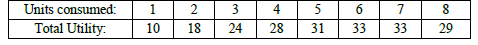# CBSE Class 12 Economics On Utility Worksheet

Download printable Economics Class 12 Worksheets in pdf format, CBSE Class 12 Economics On Utility Worksheet has been prepared as per the latest syllabus and exam pattern issued by CBSE, NCERT and KVS. Also download free pdf Economics Class 12 Assignments and practice them daily to get better marks in tests and exams for Grade 12. Free chapter wise worksheets with answers have been designed by Standard 12 teachers as per latest examination pattern

## Worksheet for Class 12 Economics On Utility

Class 12 Economics students should refer to the following printable worksheet in Pdf for On Utility in Grade 12. This test paper with questions and solutions for Standard 12 Economics will be very useful for tests and exams and help you to score better marks

### On Utility Class 12 Economics Worksheet Pdf

Worksheet on Utility

a. When marginal utility is zero, Total utility will be:
A. Increasing. B. Diminishing C. Constant D. Zero

b. When MUx/PXx > Muy/Py, Consumer will buy :
A. More units of Y and less units of X C. More units of both X and Y
B. More units of X and less units of Y D. More units of Y and same units of X

c. If a commodity is available at free of cost, how many unit will a consumer consume in order to be at equilibrium?

d. State the law of diminishing marginal utility.

e. Define utility.

SHORT ANSWER QUESTIONS (3 OR 4 Marks. Answer in around 60 to 75 words)

a. Why should a consumer buy more units of a good when its price falls? Explain in terms of utility analysis.
b. What are the assumptions of utility analysis of consumer behavior?
c. Distinguish between cardinal measurement and ordinal measurement
d. What are the limitations of utility analysis
e. Lakshmi purchases 5 units of ice cream when its price was Rs.10 per unit and is at equilibrium. Should she consume more or less to be at equilibrium when its price decreases to Rs.5 per unit? State reason.

a. How many units of a commodity should a consumer consume in order to maximize her satisfaction? Explain in terms of utility analysis.
b. A consumer consumes two goods, good X and good Y. Prices of the two goods are Px and Py respectively. Explain how consumer reaches equilibrium in the consumption of two goods.
c. Consider the following utility schedule. How many units of a commodity should a Consumer consume to be in equilibrium if market price of the good is Rs. 3 per unit? Explain with reason.## Books recommended by teachers

 CBSE Class 11 Economics Indian Economy At Eve of Independence Worksheet CBSE Class 11 Economics Indian Economy On The Eve of Independence Worksheet Set A CBSE Class 11 Economics Indian Economy On The Eve of Independence Worksheet Set B CBSE Class 11 Economics Liberalisation Privatisation And Globalisation Worksheet
 CBSE Class 11 Economics Indian Economy Worksheet Set A CBSE Class 11 Economics Indian Economy Worksheet Set B
 CBSE Class 11 Economics Poverty Worksheet
 CBSE Class 11 Economics Human Capital Formation in India Worksheet Set A CBSE Class 11 Economics Human Capital Formation in India Worksheet Set B
 CBSE Class 11 Economics Rural Development Worksheet
 CBSE Class 12 Economics Introduction To Microeconomics Worksheet CBSE Class 12 Economics Introduction to Microeconomics Worksheet CBSE Class 12 Economics Introduction Worksheet Set A CBSE Class 12 Micro Economics Introduction Worksheet
 CBSE Class 11 Economics Theory of Firm Under Perfect Competition Worksheet CBSE Class 12 Economics The Theory of Firm Under Perfect Competition Worksheet
 CBSE Class 12 Economics Market Equilibrium Worksheet
 CBSE Class 12 Economics Non Competitive Market Worksheet CBSE Class 12 Micro Economics Price Elasticity of Demand Worksheet
 CBSE Class 12 Economics Introduction To Macroeconomics Worksheet
 CBSE Class 12 Economics Money And Banking Worksheet Set A CBSE Class 12 Economics Money And Banking Worksheet Set B CBSE Class 12 Economics Money And Banking Worksheet Set C
 CBSE Class 12 Economics Determination of Income And Employment Worksheet CBSE Class 12 Economics Income Determination Worksheet
 CBSE Class 12 Economics Goverment Budget And The Economy Worksheet Set A CBSE Class 12 Economics Goverment Budget And The Economy Worksheet Set B